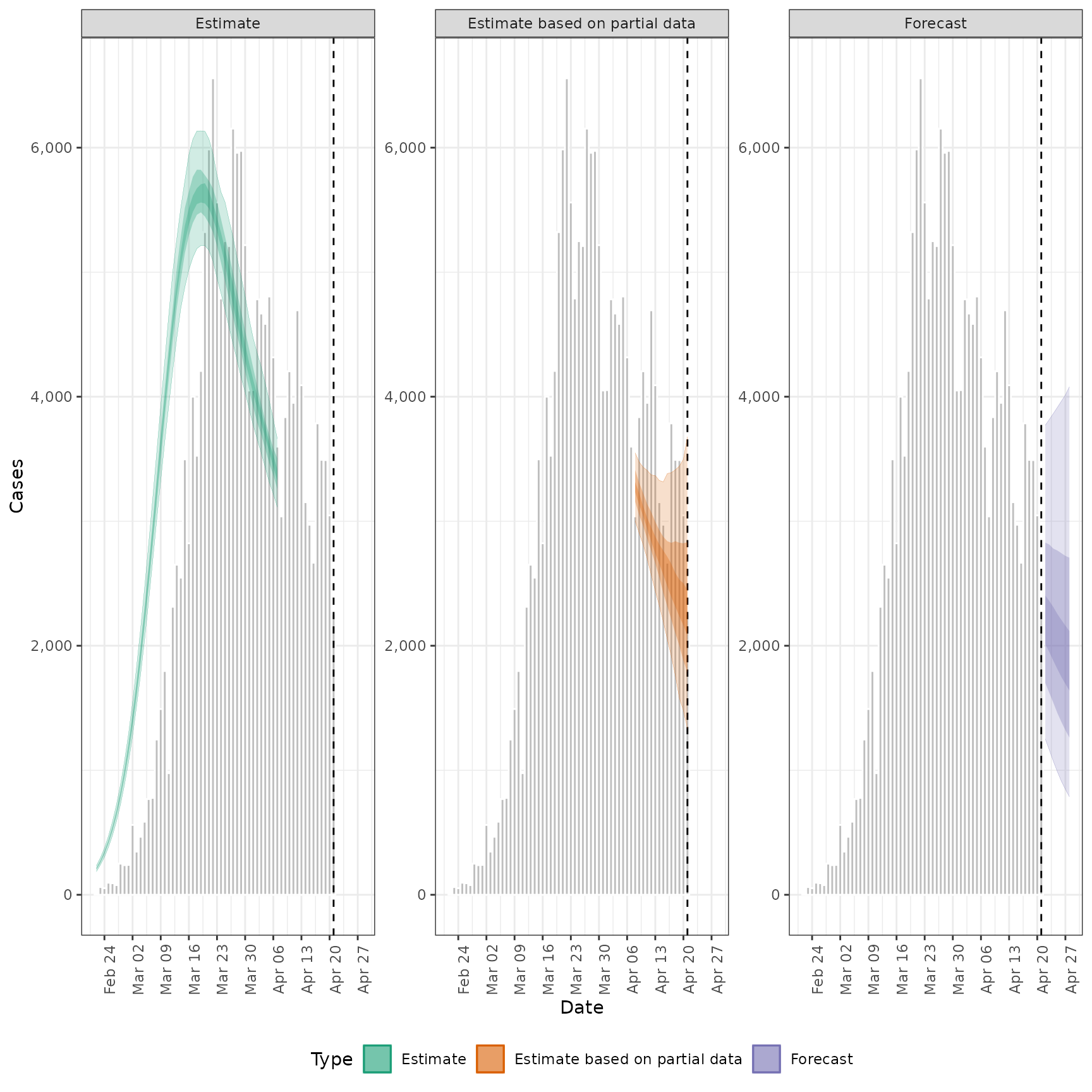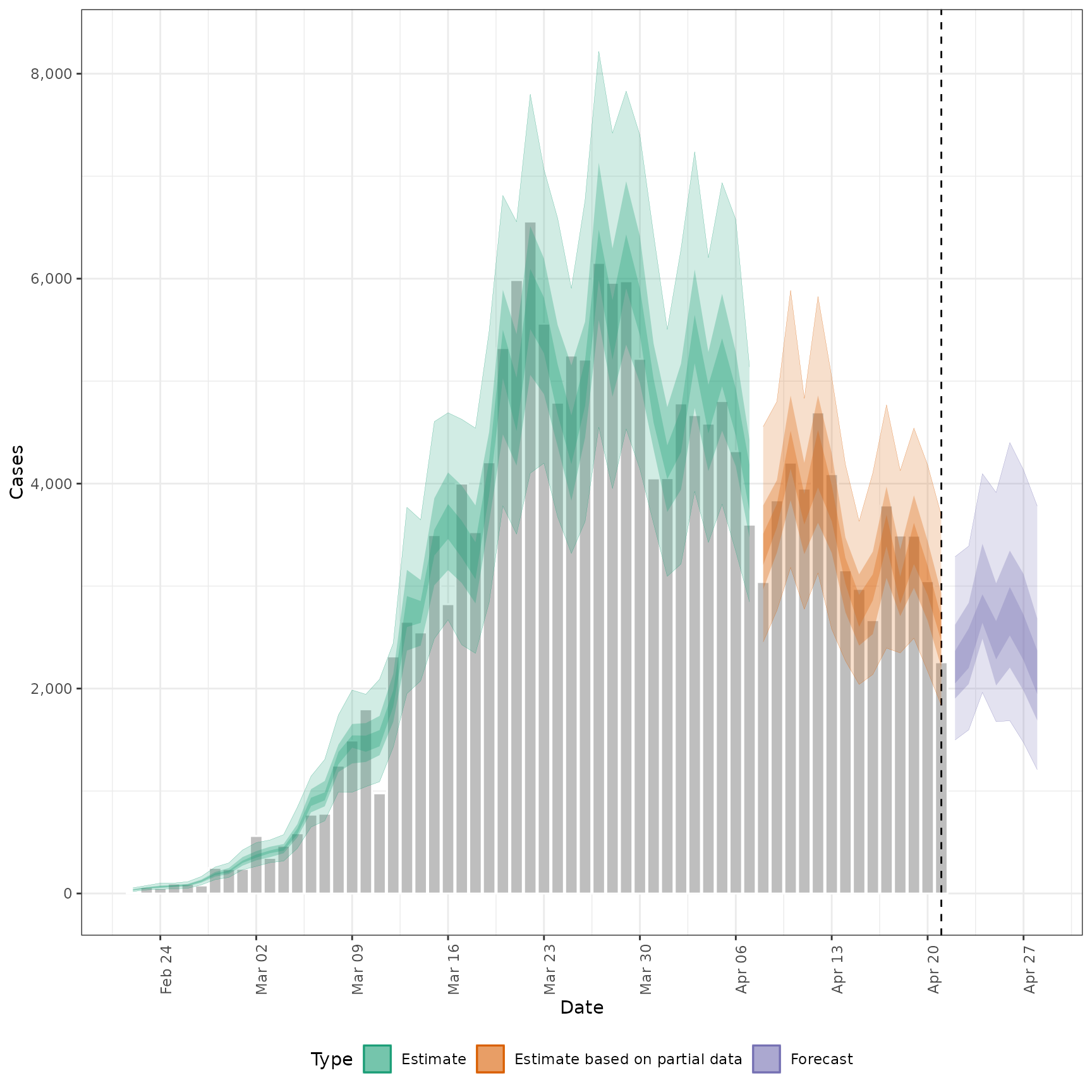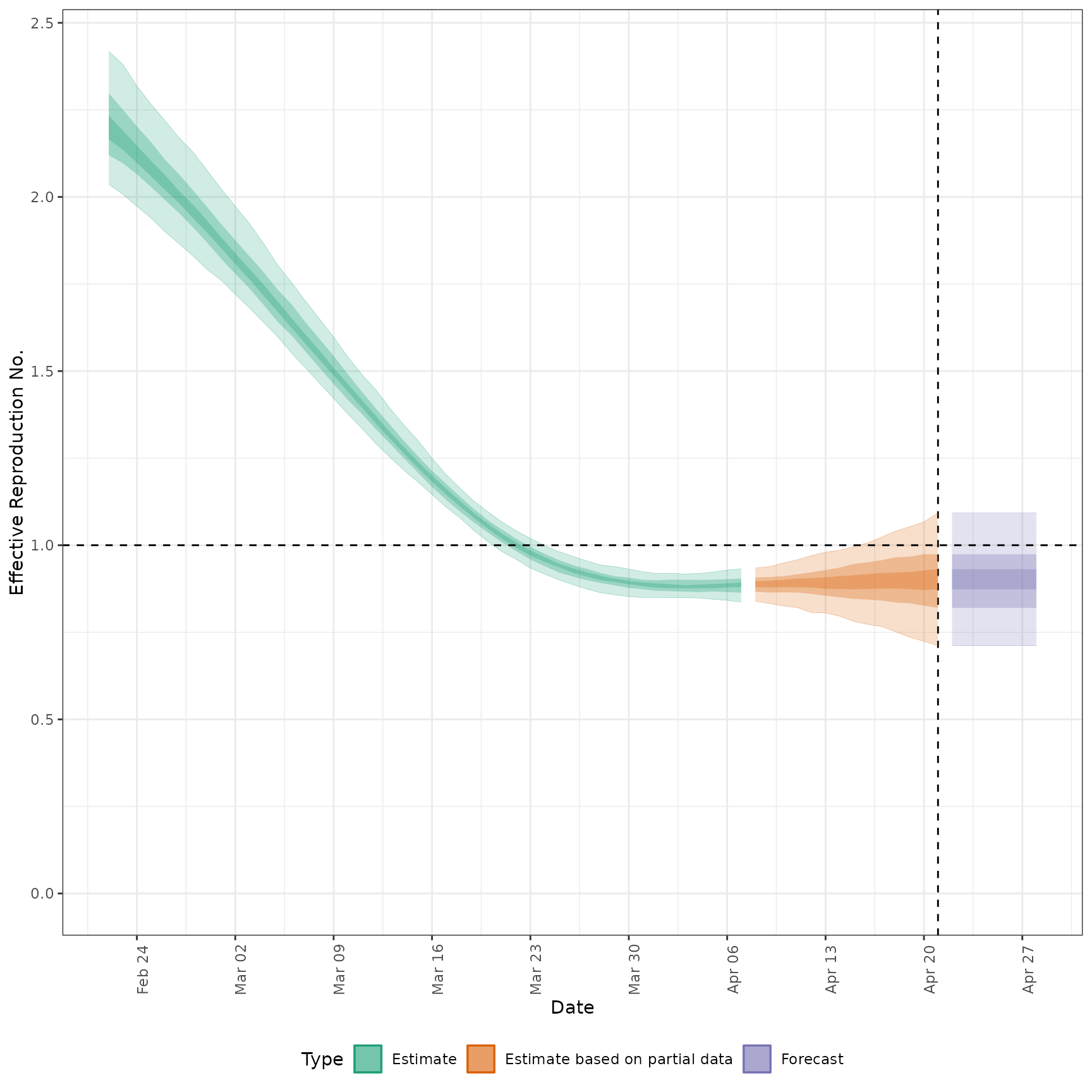Allows users to plot the output from estimate_infections easily. In future releases it may be depreciated in favour of increasing the functionality of the S3 plot methods.

plot_estimates(
estimate,
reported,
ylab = "Cases",
hline,
obs_as_col = TRUE,
max_plot = 10,
estimate_type = NULL
)

## Arguments

estimate

A data.table of estimates containing the following variables: date, type (must contain "estimate", "estimate based on partial data" and optionally "forecast"),

reported

A data.table of reported cases with the following variables: date, confirm.

ylab

Character string, defaulting to "Cases". Title for the plot y axis.

hline

Numeric, if supplied gives the horizontal intercept for a indicator line.

obs_as_col

Logical, defaults to TRUE. Should observed data, if supplied, be plotted using columns or as points (linked using a line).

max_plot

Numeric, defaults to 10. A multiplicative upper bound on the number of cases shown on the plot. Based on the maximum number of reported cases.

estimate_type

Character vector indicating the type of data to plot. Default to all types with supported options being: "Estimate", "Estimate based on partial data", and "Forecast".

## Value

A ggplot2 object

## Examples

# \donttest{
# define example cases
cases <- example_confirmed[1:40]

# set up example delays
generation_time <- get_generation_time(disease = "SARS-CoV-2", source = "ganyani")
incubation_period <- get_incubation_period(disease = "SARS-CoV-2", source = "lauer")
reporting_delay <- estimate_delay(rlnorm(100, log(6), 1), max_value = 10)

# run model
out <- estimate_infections(cases,
generation_time = generation_time,
delays = delay_opts(incubation_period, reporting_delay)
)
# plot infections
plot_estimates(
estimate = out$summarised[variable == "infections"], reported = cases, ylab = "Cases", max_plot = 2 ) + ggplot2::facet_wrap(~type, scales = "free_y")# plot reported cases estimated via Rt plot_estimates( estimate = out$summarised[variable == "reported_cases"],
reported = cases,
ylab = "Cases"
)# plot Rt estimates
plot_estimates(
estimate = out$summarised[variable == "R"], ylab = "Effective Reproduction No.", hline = 1 )#' # plot Rt estimates without forecasts plot_estimates( estimate = out$summarised[variable == "R"],
ylab = "Effective Reproduction No.",
hline = 1, estimate_type = "Estimate"
)# }